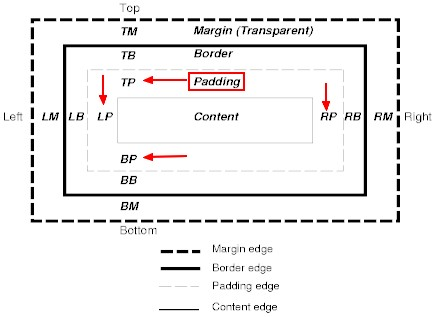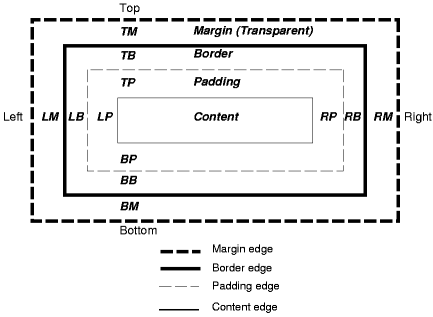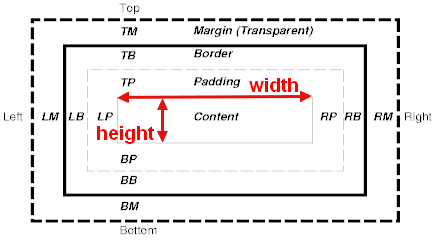# CSS - Length Value (Size)

## Introduction

Lengths refer to horizontal or vertical measurements.

## Format

The format of a length value is a number (with or without a decimal point) immediately followed by a unit identifier

````number unit`
```

where:

In sizing attribute such as width and height, there is a default unit and this is the pixel. This is also the default Svg unit

### Negative

Some properties allow negative length values, but this may complicate the formatting model and there may be implementation-specific limits. If a negative length value cannot be supported, it should be converted to the nearest value that can be supported.

If a negative length value is set on a property that does not allow negative length values, the declaration is ignored.

## Example

### Default Units on CSS and attribute

This example demonstrates that:

• the unit is mandatory in CSS (If the length does not have any unit, the property is discarded and takes the default values)
• the unit default to pixel on attribute

Example code:

``````.box {
width:10;
height:10;
}```
```
• the html with :
• a div where the css rule will apply
• an img with width and height attribute without a length of 50 without unit
``````<div class="box">
</div>```
```

</code>

``````let box = document.querySelector('.box');
let styles = window.getComputedStyle(box);
let width = styles.getPropertyValue("width");
let height = styles.getPropertyValue("height");
console.log("The computed css width is "+width);
console.log("The computed css height is "+height);```
```
``````let img = document.querySelector('img');
let imgStyles = window.getComputedStyle(img);
let widthImg = imgStyles.getPropertyValue("width");
let heightImg = imgStyles.getPropertyValue("height");
console.log("The computed img width is "+widthImg);
console.log("The computed img height is "+heightImg);```
```
• Result: we can see that:
• the css width and height are not 10 but the default values: ie
• the full available width
• a zero height.
• the img sizing has taken the default pixel unit

## Documentation / Reference

Recommended PagesThe padding area is the space between the content of the box and its border. In the below image, this are the areas: TP (top padding) BP (bottom padding) LP (left padding) RB (right padding)...CSS - (Length) Unit

CSS CSS The unit in CSS is the second element of a length that defines the type of the length number. Example: px, em, etc ... The recommended unit scales are: Screen: rem, em, px, % ...CSS - Height of a box

This page is the height of a box. From How it's calculated To How it's defined HTMLheight attributeHTMLimage...div element When a absolute length value is set, the value applied directly...
CSS - Left property explained as 1, 2, 3

The left property is an offset from the left edge of the containing block. This article shows you in two simple rules how to determine the containing block.
CSS - Percentage Value

The format of a percentage value is a number followed by the character %. Percentage values are always relative to another value, for example a length. Each property that allows percentages also defines...
CSS - Print

Print is a media type Intended for paged material and for documents viewed on screen in print preview mode. When creating stylesheet for this media, the point unit is generally used for length definition...CSS - Relative Sizing (Length)

Relative Sizing is when you are using a length units that is relative to another length property. Style sheets that use relative units will more easily scale from one medium to another (e.g., from a...CSS - Rem (Root Emphemeral Unit)

CSS rem is a length unit that is based in the the font-size of the root element of the document. To write style rules that is constant throughout the document, use the rem rem stands for root em...
CSS - Top Property

The top property is a offset property that specifies how far is positioned the box from the top edge of its . It applies only if the box itself has a position value of: relative absolute or fixed...CSS - Width property (of a box)

CSS The width property specifies the width of boxes and it's calculation is defined by the box sizing property. HTMLCSS HTML width attributeHTMLimgiframe... If you want to set a width on a p element...